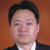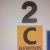# mongo.NumberInt(`\${record[key]}`) throws an error when record[key] = 10004743968

Voir Hillaire shared this problem ago
Solved

`val = _.isInteger(record[key]) ? NumberInt(`\${record[key]}`) : record[key];`
_.isInteger() should check for out of range so large numbers can be double. As a work-around I wrapped the code in a try/catch:

```            for (let key in record) {
let val =  record[key];
try {

val = _.isInteger(record[key]) ? NumberInt(`\${record[key]}`) : record[key];
}
catch(err){
//console.log(err);
}
dst[fieldMap[key] || key] = val;
}
```2

Thank you for your feedback. It 's a bug. We have worked out a new test build to resolve the issue. Please re-download and try it again.

Here is the modified code

```            for (let key in record) {
const val = ((v) => {
if (_.isInteger(v)) {
if (v > 2147483647 || v < -2147483648) {
return NumberLong(`\${v}`); //convert to int64
} else {
return NumberInt(`\${v}`)
}
} else {
return v;
}
})(record[key]);

dst[fieldMap[key] || key] = val;
}```1

That works, thank you very much